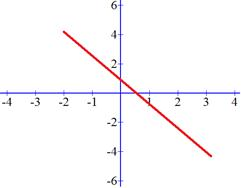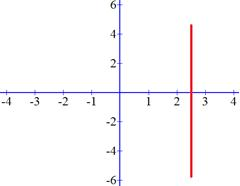### Sample Problem

A.B.C.D.Which graph has:

1) Positive Slope?

2) Negative Slope?

3) Zero Slope?

4) Undefined Slope?

(Enter the letter of the graph)

#### Solution

1) Graph A increases from left to right, so it is increasing.

2) Graph C decreases from left to right, so it is decreasing.

3) Graph B stays constant from left to right, so it has zero slope.

4) Graph D is a vertical line, a rise without a run, so it has undefined slope.